# How to Calculate Depreciation on Fixed Assets

Depreciation is the method of calculating the cost of an asset over its lifespan. Calculating the depreciation of a fixed asset is simple once you know the formula.# Enter the asset’s purchase price.

For example, if you bought factory equipment for \$1,000, then that’s the amount that you’ll use as the purchase price.# Subtract the salvage value from the purchase price to find the depreciable cost.

The “scrap” or “salvage” value of the item represents how much it will be worth once it’s outlived its usefulness. Subtract that number from the purchase price to get the depreciable cost.
For example, if you bought the factory equipment mentioned above for \$1,000 and determined that it would be worth only \$200 at the end of its lifespan, then the depreciable cost is \$1,000 – \$200 or \$800.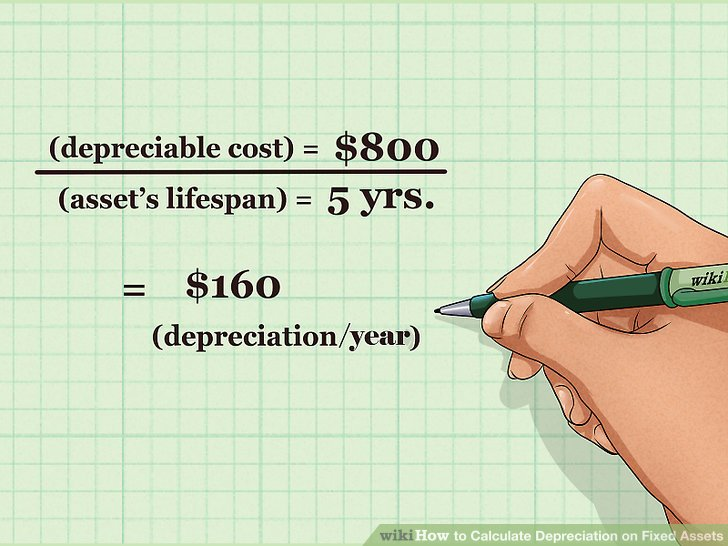# Divide the depreciable cost by the asset’s lifespan to get the depreciation.

The asset that you purchased has an expected lifespan just like anything else (your personal computer, for example, is something that you probably don’t expect to use for more than a few years). You’ll need to know how many years you can expect to get any use out of your new asset and then divide the depreciable cost by that number.
For example, if the depreciable value of the asset is \$800 and you expect it to last 5 years, then the depreciation is \$800 / 5 = \$160. That’s the amount of depreciation for the asset that you’ll enter in your accounting books every year.# Determine the expected lifespan of the asset.

Your fixed asset has a lifespan, after which it will no longer be of use. That number is usually measured in years. In this example, assume that you purchased factory equipment for \$1,000, you expect it to last five years, and that it has a salvage value of \$200.# Divide 100% by the number of years in the asset life and then multiply by 2 to find the depreciation rate.

Remember, the factory equipment is expected to last five years, so this is how your calculations would look: 100% / 5 years = 20% and 20% x 2 = 40%.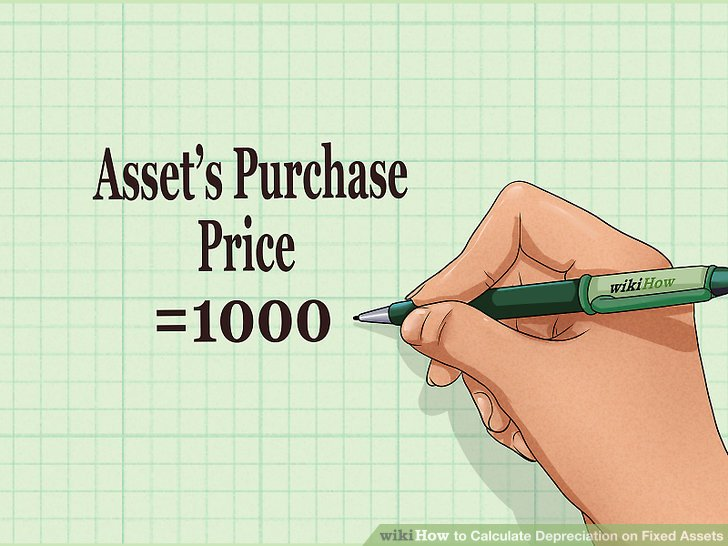# Determine the asset’s purchase price.

In this example, the asset was purchased for \$1,000.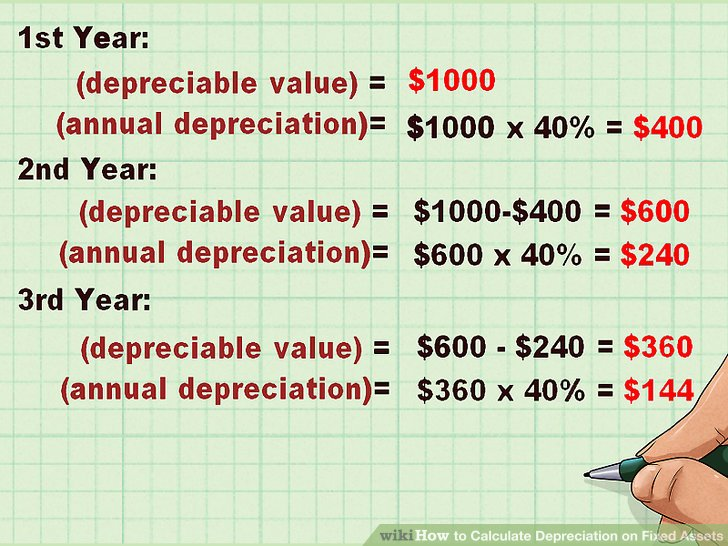# Multiply the current value of the asset by the depreciation rate.

This calculation will give you a different depreciation amount every year.

In the first year of use, the depreciation will be \$400 (\$1,000 x 40%).
For the second year, the depreciable cost is now \$600 (\$1,000 – \$400 depreciation from the previous year) and the annual depreciation will be \$240 (\$600 x 40%).
For the third year, the depreciable cost becomes \$360 with a depreciation of \$144, and so on.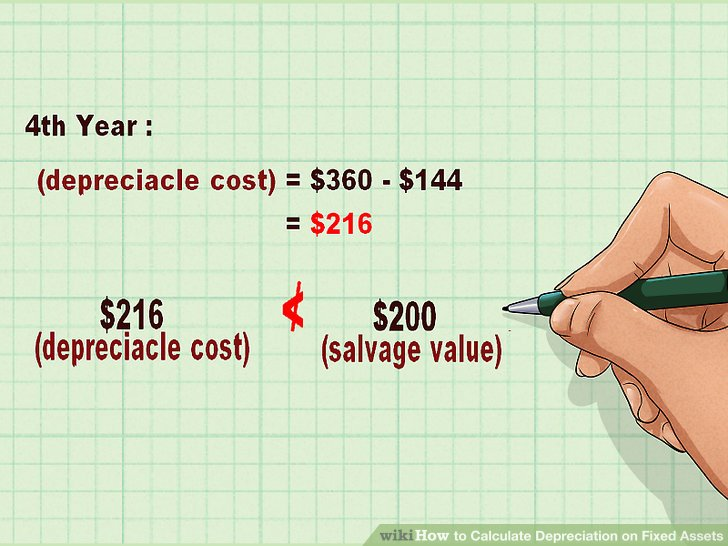# Stop accumulating depreciation in any year in which the depreciable cost falls below the salvage value.

Using this example, in year 4 the depreciable cost is \$216. The salvage value is \$200.
In year 4, calculate depreciation of \$16 to reduce the final value to \$200.
In year 5, there is no need to calculate depreciation.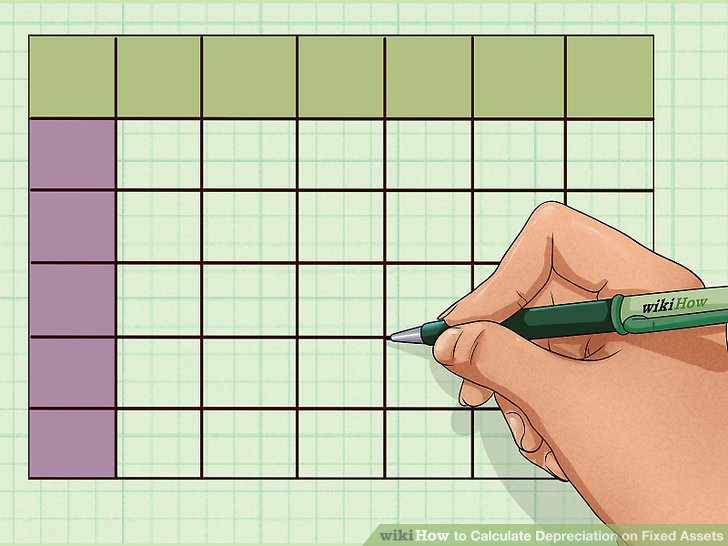This will be in table format and it’s great to use a spreadsheet for this type of calculation.
Your table should have 6 columns and one row for each year in the asset’s life plus a header row.# Label the columns in the header row.

Use the following headers: Beginning Book Value, Total Depreciable Cost, Depreciation Rate, Depreciation Expense, Accumulated Depreciation and Ending Book Value.# Enter the purchase price of the asset in the first row under the Beginning Book Value column.

As an example, assume a purchase price of \$1,000 with a lifespan of five years and a salvage value of \$200.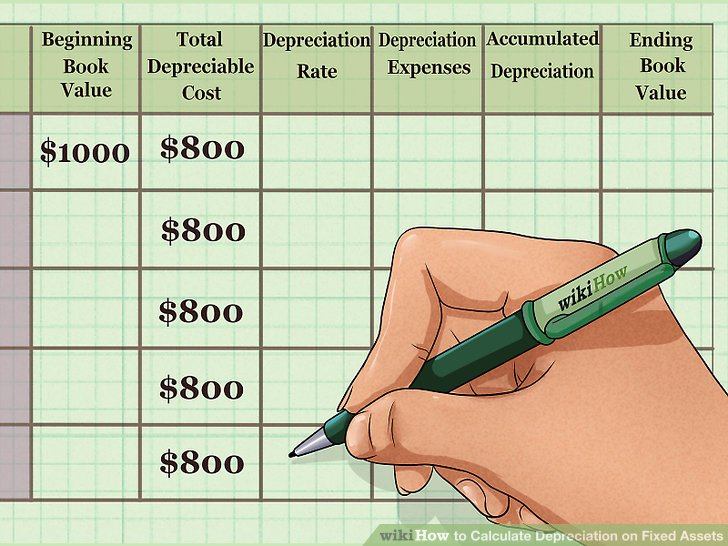# Subtract the salvage value, if any, from the original cost and enter this number in all rows under the Total Depreciable Cost column.

In this example, the value is \$1,000 purchase price minus \$200 salvage value equals \$800 depreciable cost.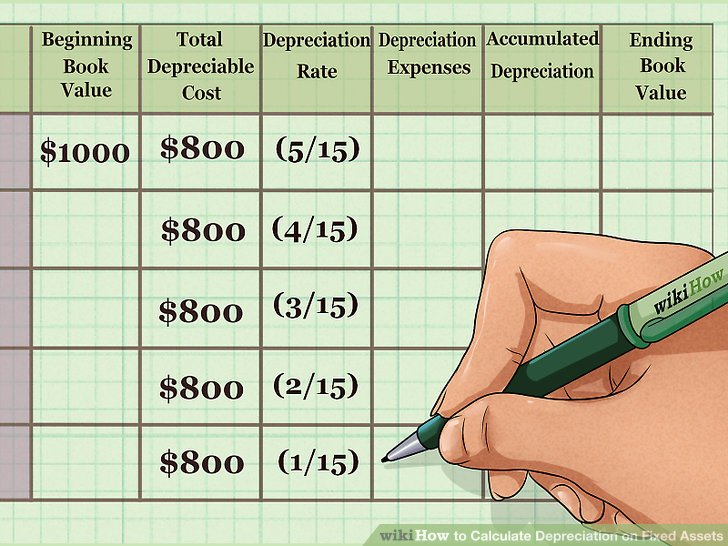# Calculate the depreciation rate.

As the method name implies, you’ll do this by summing up the years.

Sum the numbers of the years in the asset’s depreciable life. Using the example of 5 years, that would be 15 (1 + 2 + 3 + 4 + 5 = 15).
In the first year, divide the sum by the last number (5 / 15); in the second year the sum is divided by the second-to-last number (4 / 15) and so on down the column to find the percentage of depreciation rate for each year.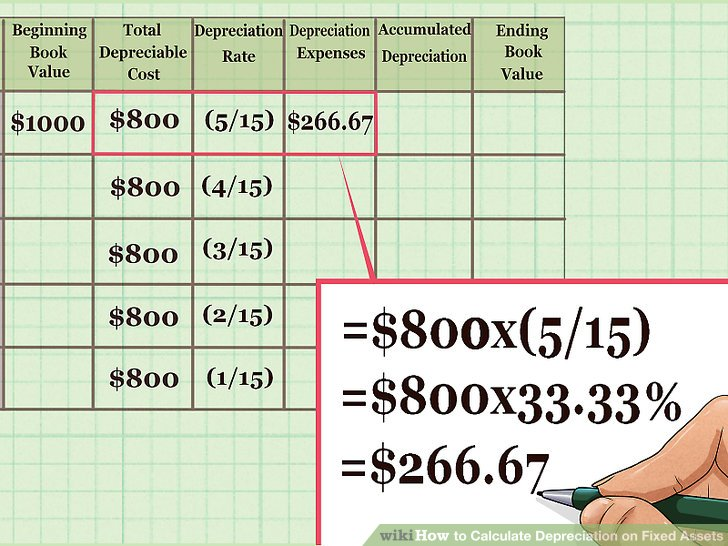# Find the depreciation expense by multiplying the depreciable cost in the first year by the depreciation rate.

You’ll use the sum you derived above to reach this value.
\$800 x (5/15) = \$800 x 33.33 percent = \$266.67.# Subtract the first year’s deprecation from the Beginning Book Value.

That number will give you the Ending Book Value for that year. It will also be the second year’s Beginning Book Value.
For example: \$1,000 – \$266.67 = \$733.33.# Fill in the rest of the schedule.

You’ll follow the same pattern with the subsequent years, using the beginning book value for each year as the starting point and using the appropriate percentage based on the year (4 / 15 in the second year, 3 /15 in the third year, etc.).
In year 2, the depreciation amount is \$213.33 (\$733.33 x 26.67%); in year 3 it is \$160 (\$520 x 20%); in year 4 it is \$106.67 (\$360 x 13.3%) and in year 5 it is \$53.33 (253.33 x 6.67%).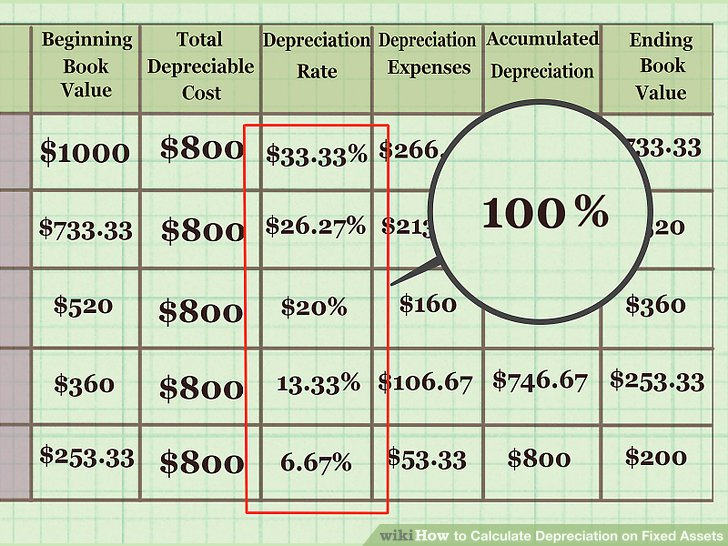# Check your math at the end of the schedule.

All the percentages in the Depreciation Rate column will total 100 percent. The Accumulated Depreciation will equal the purchase price less the salvage value (in this case, \$200).

Categories:   Youth